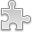# Courses / Module

Toggle Print

##MATHEMATICAL BIOLOGY

Module code: MT481S
Credits: 5
Semester: 2
Department: MATHEMATICS AND STATISTICS
International:Overview

Module Objective:

To demonstrate how Mathematics can be used in Biology.

Single species models, continuous and discrete models, exponential, logistic and difference equation models. Steady states and stability, introduction to qualitative methods. Models for interacting species, Lotka-Volterra equations, qualitative behaviour of solutions of linear systems, predator-prey systems, harvesting. Structured population models, epidemic models. Deterministic modelling (ordinary differential equations and difference equations).Learning OutcomesTeaching & Learning methodsAssessmentAutumn Supplementals/ResitsPre-RequisitesTimetable